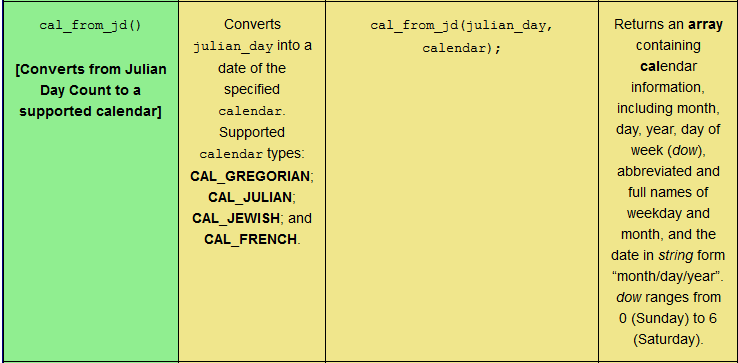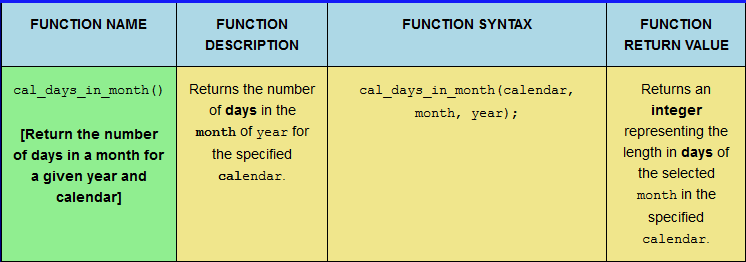# PHP Calendar Function Quiz: `jdmonthname()`; `jdtofrench()`; `jdtogregorian()`; `jdtojewish()`; `jdtojulian()`; `jdtounix()`; `jewishtojd()`; `juliantojd()`; and `unixtojd()`.This post serves as a reference point for 9 PHP calendar functions: `jdmonthname()`; `jdtofrench()`; `jdtogregorian()`; `jdtojewish()`; `jdtojulian()`; `jdtounix()`; `jewishtojd()`; `juliantojd()`; and `unixtojd()`. Users can also click a ‘RANDOMIZE’ button, whereupon all the function descriptions, function syntaxes, and function return values are randomized. The user can then rearrange the table cells correctly (thus completing the quiz) or click an ‘AUTO COMPLETE’ button (following further optional manual randomization) and watch the computer complete the quiz itself! The computer completes the quiz at a speed that the human eye can follow, thus aiding learning.

# PHP Calendar Function Quiz: `cal_days_in_month()`; `cal_from_jd()`; `cal_info()`; `cal_to_jd()`; `easter_date()`; `easter_days()`; `frenchtojd()`; `gregoriantojd()`; and `jddayofweek()`.This post serves as a reference point for 9 PHP calendar functions: `cal_days_in_month()`; `cal_from_jd()`; `cal_info()`; `cal_to_jd()`; `easter_date()`; `easter_days()`; `frenchtojd()`; `gregoriantojd()`; and `jddayofweek()`. Users can also click a ‘RANDOMIZE’ button, whereupon all the function descriptions, function syntaxes, and function return values are randomized. The user can then rearrange the table cells correctly (thus completing the quiz) or click an ‘AUTO COMPLETE’ button (following further optional manual randomization) and watch the computer complete the quiz itself! The computer completes the quiz at a speed that the human eye can follow, thus aiding learning.

# PHP Quiz: All Calendar FunctionsThis post serves as a reference point for 18 PHP calendar functions. Users can also click a ‘RANDOMIZE’ button, whereupon all the function descriptions, function syntaxes, and function return values are randomized. The user can then rearrange the table cells correctly (thus completing the quiz) or click an ‘AUTO COMPLETE’ button (following further optional manual randomization) and watch the computer complete the quiz itself! The computer completes the quiz at a speed that the human eye can follow, thus aiding learning.